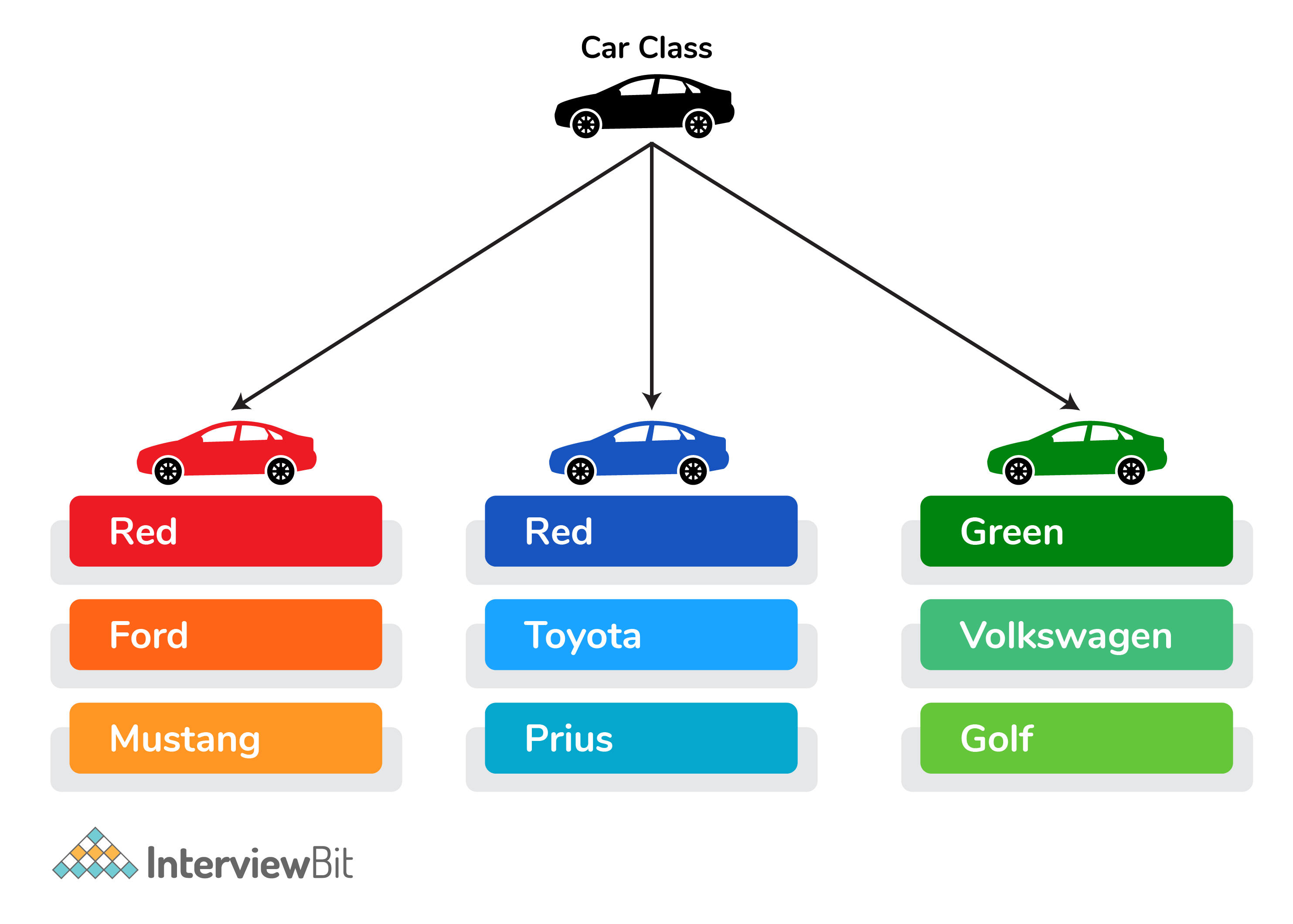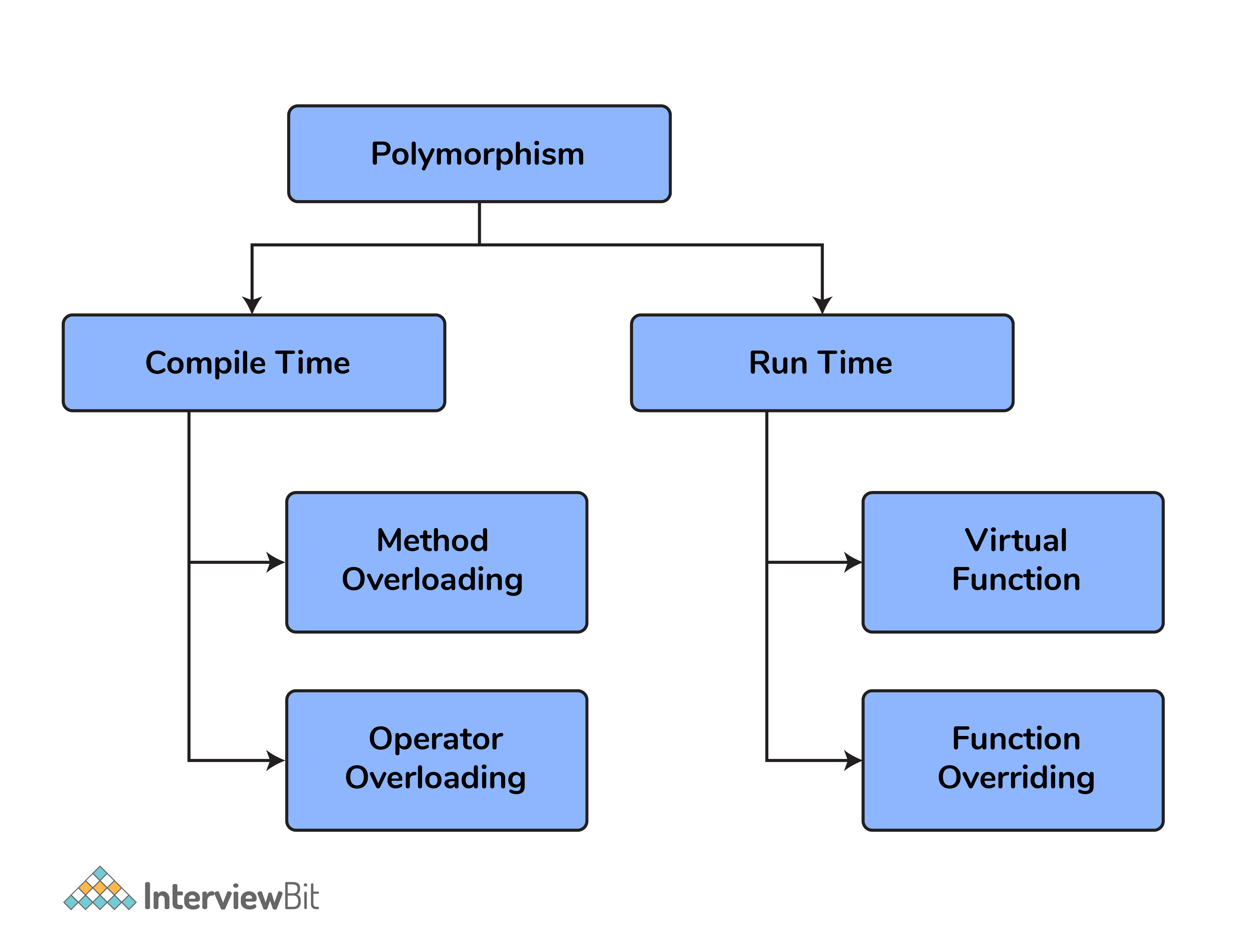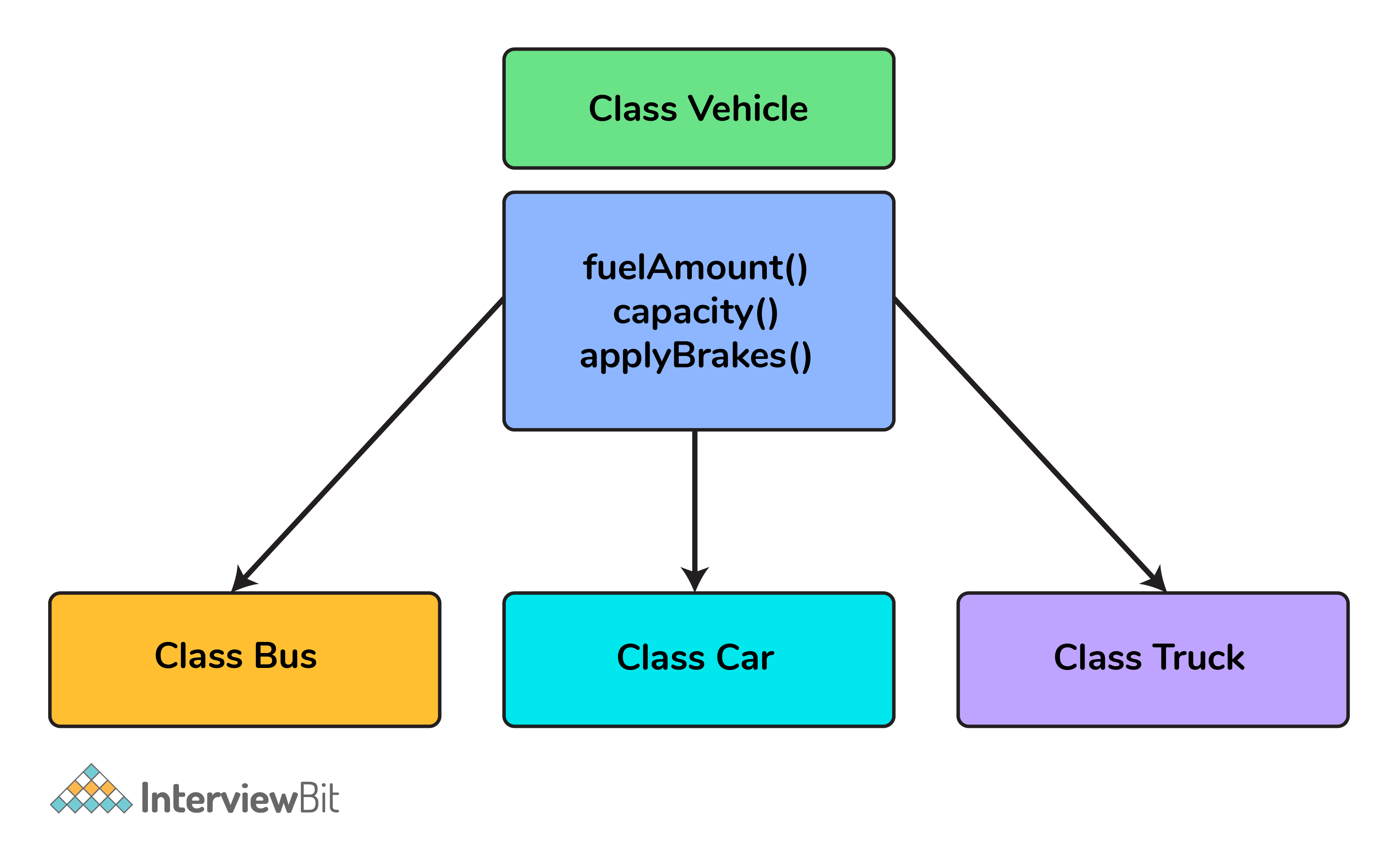## Welcome to Interviewbit, help us create the best experience for you!

Currently, You are a:## Few details about your education

College/University *
Enter the name of your college
Branch *
Year of completion *## Few details about your education

College/University *
Enter the name of your college
Branch *
Year of completion *## Few details about your career...

Current Company *
Enter company name
Experience *## You're all set!## Begin your success journey!

Sign Up using
Full name *
Email *
Password *

By creating an account, I acknowledge that I have read and agree to InterviewBit’s Terms and Privacy Policy .Log In using
Email *
Password *

# C++ Interview Questions

### Download PDF

Your requested download is ready!
Click here to download.## Begin your success journey!

Sign Up using
Full name *
Email *
Password *

By creating an account, I acknowledge that I have read and agree to InterviewBit’s Terms and Privacy Policy .## Welcome back!

Log In using
Email *
Password *

C++ is a powerful and all-purpose programming tool developed by Bjarne Stroustrup at Bell Labs. This language is an extension of C and is by far one of the fastest object-oriented programming languages. C++ is super popular because of its high speed and compatibility.

It is widely used in the development of games and servers while some of the real-world applications of C++ are as follows

• Operating systems
• GUI based applications
• Distributed systems
• Database software
• Banking applications
• Advanced computations and graphics
• Embedded systems

So, today, well understand the different C++ questions asked in an interview at a basic, intermediate and advanced level.

## C++ Interview Questions For Freshers

### 1. What are the different data types present in C++?

The 4 data types in C++ are given below:

• Primitive Datatype(basic datatype). Example- char, short, int, float, long, double, bool, etc.
• Derived datatype. Example- array, pointer, etc.
• Enumeration. Example- enum
• User-defined data types. Example- structure, class, etc.

### 2. What is the difference between C and C++?

The main difference between C and C++ are provided in the table below:

### 3. What are class and object in C++?

A class is a user-defined data type that has data members and member functions. Data members are the data variables and member functions are the functions that are used to perform operations on these variables.

An object is an instance of a class. Since a class is a user-defined data type so an object can also be called a variable of that data type.

A class is defined as-

``````class A{
private:
int data;
public:
void fun(){

}
};``````Class and Object in C++

For example, the following is a class car that can have properties like name, color, etc. and they can have methods like speed().

### 4. What is the difference between struct and class?

In C++ a structure is the same as a class except for a few differences like security. The difference between struct and class are given below:

### 5. What is operator overloading?

Operator Overloading is a very essential element to perform the operations on user-defined data types. By operator overloading we can modify the default meaning to the operators like +, -, *, /, <=, etc.

For example -

The following code is for adding two complex number using operator overloading-

``````class complex{
private:
float r, i;
public:
complex(float r, float i){
this->r=r;
this->i=i;
}
complex(){}
void displaydata(){
cout<<”real part = “<<r<<endl;
cout<<”imaginary part = “<<i<<endl;
}
complex operator+(complex c){
return complex(r+c.r, i+c.i);
}
};
int main(){
complex a(2,3);
complex b(3,4);
complex c=a+b;
c.displaydata();
return 0;
}``````

### 6. What is polymorphism in C++?

Polymorphism in simple means having many forms. Its behavior is different in different situations. And this occurs when we have multiple classes that are related to each other by inheritance.

For example, think of a base class called a car that has a method called car brand(). Derived classes of cars could be Mercedes, BMW, Audi - And they also have their own implementation of a cars

The two types of polymorphism in c++ are:

• Compile Time Polymorphism
• Runtime PolymorphismPolymorphism in C++

### 7. Explain constructor in C++

The constructor is a member function that is executed automatically whenever an object is created. Constructors have the same name as the class of which they are members so that compiler knows that the member function is a constructor. And no return type is used for constructors.

Example:

``````class A{
private:
int val;
public:
A(int x){             //one argument constructor
val=x;
}
A(){                    //zero argument constructor
}
}
int main(){
A a(3);

return 0;
}``````

### 8. Tell me about virtual function

Virtual function is a member function in the base class that you redefine in a derived class. A virtual function is declared using the virtual keyword. When the function is made virtual, C++ determines which function is to be invoked at the runtime based on the type of the object pointed by the base class pointer.

### 9. Compare compile time polymorphism and Runtime polymorphism

The main difference between compile-time and runtime polymorphism is provided below:

### 10. What do you know about friend class and friend function?

A friend class can access private, protected, and public members of other classes in which it is declared as friends.

Like friend class, friend function can also access private, protected, and public members. But, Friend functions are not member functions.

For example -

``````class A{
private:
int data_a;
public:
A(int x){
data_a=x;
}
friend int fun(A, B);
}
class B{
private:
int data_b;
public:
A(int x){
data_b=x;
}
friend int fun(A, B);
}
int fun(A a, B b){
return a.data_a+b.data_b;
}
int main(){
A a(10);
B b(20);
cout<<fun(a,b)<<endl;
return 0;
}``````

Here we can access the private data of class A and class B.

### 11. What are the C++ access specifiers?

In C++ there are the following access specifiers:

Public: All data members and member functions are accessible outside the class.

Protected: All data members and member functions are accessible inside the class and to the derived class.

Private: All data members and member functions are not accessible outside the class.

### 12. Define inline function

If a function is inline, the compiler places a copy of the code of that function at each point where the function is called at compile time. One of the important advantages of using an inline function is that it eliminates the function calling overhead of a traditional function.

### 13. What is a reference in C++?

A reference is like a pointer. It is another name of an already existing variable. Once a reference name is initialized with a variable, that variable can be accessed by the variable name or reference name both.

For example-

``````int x=10;
int &ref=x;           //reference variable``````

If we change the value of ref it will be reflected in x. Once a reference variable is initialized it cannot refer to any other variable. We can declare an array of pointers but an array of references is not possible.

### 14. What do you mean by abstraction in C++?

Abstraction is the process of showing the essential details to the user and hiding the details which we don’t want to show to the user or hiding the details which are irrelevant to a particular user.

### 15. Is deconstructor overloading possible? If yes then explain and if no then why?

No destructor overloading is not possible. Destructors take no arguments, so there’s only one way to destroy an object. That’s the reason destructor overloading is not possible.

### 16. What do you mean by call by value and call by reference?

In call by value method, we pass a copy of the parameter is passed to the functions. For these copied values a new memory is assigned and changes made to these values do not reflect the variable in the main function.

In call by reference method, we pass the address of the variable and the address is used to access the actual argument used in the function call. So changes made in the parameter alter the passing argument.

### 17. What is an abstract class and when do you use it?

A class is called an abstract class whose objects can never be created. Such a class exists as a parent for the derived classes. We can make a class abstract by placing a pure virtual function in the class.

### 18. What are destructors in C++?

A constructor is automatically called when an object is first created. Similarly when an object is destroyed a function called destructor automatically gets called. A destructor has the same name as the constructor (which is the same as the class name) but is preceded by a tilde.

Example:

``````class A{
private:
int val;
public:
A(int x){
val=x;
}
A(){
}
~A(){                  //destructor
}
}
int main(){
A a(3);
return 0;
}``````

### 19. What are the static members and static member functions?

When a variable in a class is declared static, space for it is allocated for the lifetime of the program. No matter how many objects of that class have been created, there is only one copy of the static member. So same static member can be accessed by all the objects of that class.

A static member function can be called even if no objects of the class exist and the static function are accessed using only the class name and the scope resolution operator ::

### 20. Explain inheritance

Inheritance is the process of creating new classes, called derived classes, from existing classes. These existing classes are called base classes. The derived classes inherit all the capabilities of the base class but can add new features and refinements of their own.

Example-Inheritance in C++

Class Bus, Class Car, and Class Truck inherit the properties of Class Vehicle.

The most important thing about inheritance is that it permits code reusability.

## C++ Interview Questions For Experienced

### 21. What is a copy constructor?

A copy constructor is a member function that initializes an object using another object of the same class.

Example-

``````class A{
int x,y;
A(int x, int y){
this->x=x;
this->y=y;
}

};
int main(){
A a1(2,3);
A a2=a1;     //default copy constructor is called
return 0;
}``````

We can define our copy constructor. If we don’t define a copy constructor then the default copy constructor is called.

### 22. What is the difference between shallow copy and deep copy?

The difference between shallow copy and a deep copy is given below:

### 23. What is the difference between virtual functions and pure virtual functions?

A virtual function is a member function in the base class that you redefine in a derived class. It is declared using the virtual keyword.

Example-

``````class base{
public:
virtual void fun(){

}
};``````

A pure virtual function is a function that has no implementation and is declared by assigning 0. It has no body.

Example-

``````class base{
public:
virtual void fun()=0;
};``````

Here, = sign has got nothing to do with the assignment, and value 0 is not assigned to anything. It is used to simply tell the compiler that a function will be pure and it will not have anybody.

### 24. If class D is derived from a base class B. When creating an object of type D in what order would the constructors of these classes get called?

The derived class has two parts, a base part, and a derived part.  When C++ constructs derived objects, it does so in phases. First, the most-base class(at the top of the inheritance tree) is constructed. Then each child class is constructed in order until the most-child class is constructed last.
So the first Constructor of class B will be called and then the constructor of class D will be called.

During the destruction exactly reverse order is followed. That is destructor starts at the most-derived class and works its way down to base class.
So the first destructor of class D will be called and then the destructor of class B will be called.

### 25. Can we call a virtual function from a constructor?

Yes, we can call a virtual function from a constructor. But the behavior is a little different in this case. When a virtual function is called, the virtual call is resolved at runtime. It is always the member function of the current class that gets called. That is the virtual machine doesn’t work within the constructor.

For example-

``````class base{
private:
int value;
public:
base(int x){
value=x;
}
virtual void fun(){

}
}

class derived{
private:
int a;
public:
derived(int x, int y):base(x){
base *b;
b=this;
b->fun();      //calls derived::fun()
}
void fun(){
cout<<”fun inside derived class”<<endl;
}
}
``````

### 26. What are void pointers?

A void pointer is a pointer which is having no datatype associated with it. It can hold addresses of any type.

For example-

``````void *ptr;
char *str;
p=str;                // no error
str=p;                // error because of type mismatch``````

We can assign a pointer of any type to a void pointer but the reverse is not true unless you typecast it as

``str=(char*) ptr;``

### 27. What is this pointer in C++?

The member functions of every object have a pointer named this, which points to the object itself. The value of this is set to the address of the object for which it is called. It can be used to access the data in the object it points to.

Example

``````class A{
private:
int value;
public:
void setvalue(int x){
this->value=x;
}
};

int main(){
A a;
a.setvalue(5);
return 0;
}``````

### 28. How do you allocate and deallocate memory in C++?

The new operator is used for memory allocation and deletes operator is used for memory deallocation in C++.

For example-

``````int value=new int;  		//allocates memory for storing 1 integer
delete value;          		// deallocates memory taken by value

int *arr=new int;    	//allocates memory for storing 10 int
delete []arr;              	// deallocates memory occupied by arr``````

Additional Resources

Practice Coding

C++ Tutorials

C Interview Questions

## C++ Coding Questions

1.

Which operator can not be overloaded in C++?

2.

What will be the output of the following C++ program:

``````#include<iostream>
using namespace std;
int main(){
int a=1;
cout<<(a++)*(++a)<<endl;
return 0;
}``````
3.

What will be the value of x in the following C++ program

``````#include<iostream>
using namespace std;
int main(){
int a=1;
int x=(a++)++;
cout<<x<<endl;
return 0;
}``````
4.

What is an abstract class?

5.

Consider the following C++ program

``````#include<iostream>
using namespace std;
class A{
public:
virtual void a()=0;
A(){
cout<<"A ";
}
};
class B: public A
{
public:
B(){
cout<<"B ";
}
};

int main(){
A *a=new B();

return 0;
}``````

What will be output?

6.

What is the size of void in C++?

7.

If a base class and derived class each include a member function with the same name. Function from which class will be called if called by an object of the derived class

8.

Memory used by an array is

9.

Which of the following statement is correct?